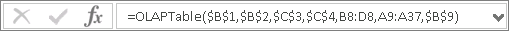OLAPTableThe OLAPTable function is the default setting and is the most commonly used setting for creating an Excel worksheet. The OLAPTable setting means that when creating/updating your worksheet, PowerOLAP sends the data in table form, as an array of Fact Data, as opposed to cell by cell. The OLAPTable reference formula is in a cell outside of the array of Fact Data. In the first exercise in this section, we saw that the cell reference formula in cell A7 of the Excel slice was an OLAPTable reference. It looked like this in the formula bar:This setting speeds recalculation time, especially in large tables, but does not give you the flexibility of the next option, OLAPReadWrite. For example, you can not insert blank rows and columns into the array; nor can you use an Excel formula within a cell in the array.